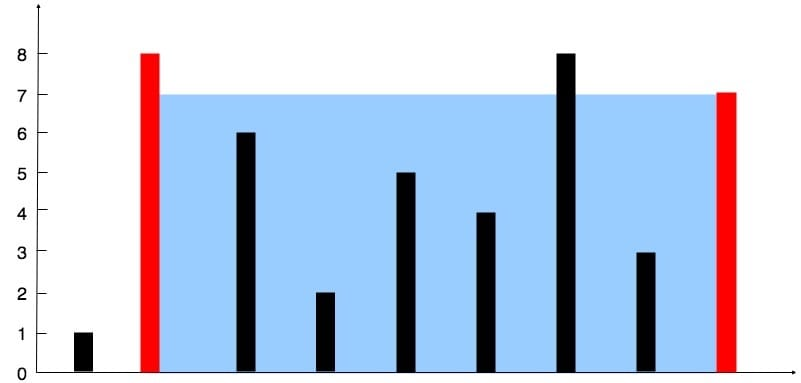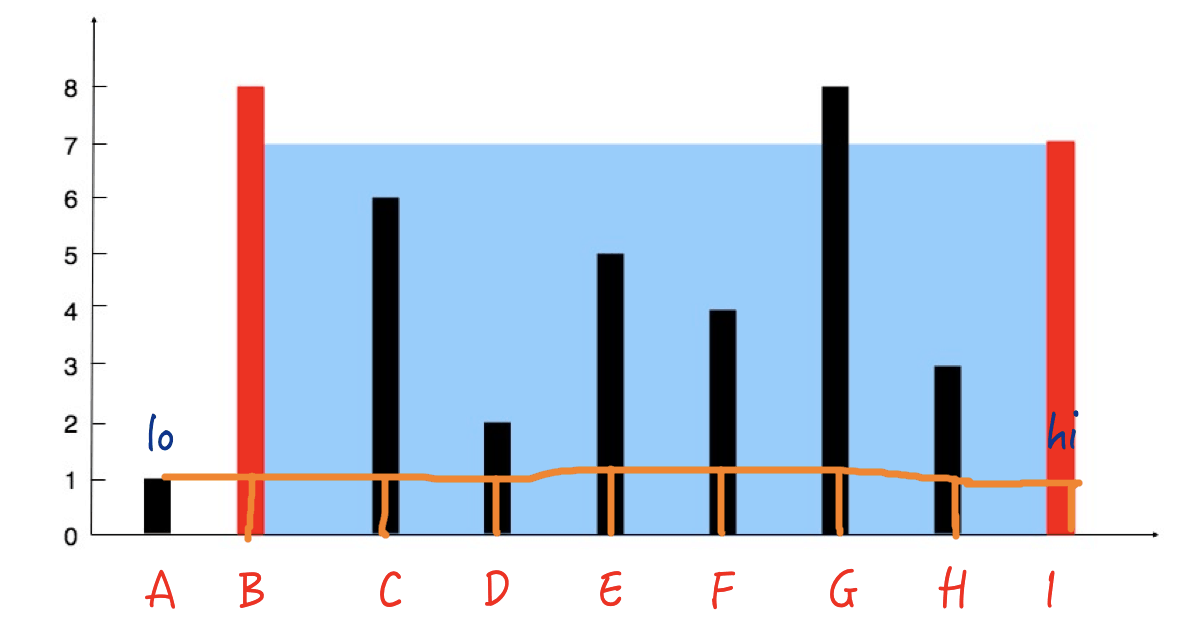# 11. Container With Most Water

Reference: LeetCode
Difficulty: Medium

## Problem

Given n non-negative integers a1, a2, ..., an, where each represents a point at coordinate (i, ai). n vertical lines are drawn such that the two endpoints of line i is at (i, ai) and (i, 0). Find two lines, which together with x-axis forms a container, such that the container contains the most water.Note: You may not slant the container and n is at least 2.

Example:

## Analysis

### Brute-Force

Try every container.

Time: $O(N^2)$
Space: $O(1)$

### Two Pointers

We have two pointers lo and hi that start from two ends. Each time we update the one with smaller height.

Why?

It is kind of pruning unnecessary cases. If height[lo] < height[hi], we move lo because we know that the areas in which lo is the left bar ([lo, lo + 1],[lo, lo + 2], …, [lo, hi - 1]) must be less than the area in [lo, hi] that we just considered. So next time we should not consider lo as the left end anymore.For example, all the areas (AB, AC, AD, …, AH) are less than AI.

Time: $O(N)$
Space: $O(1)$

CommentJunhao Wang
a software engineering cat